CPSC536A - Notes for Class 8 - Multiple Sequence Alignment - continued

Jan 30, 2001

Last lecture: We look for an almost optimal solution -> need polynomial time instead of exponential

Today: How it is done in practice?

Progressive / Iterative Multiple Sequence Alignment (Progressive / Iterative MSA)

Give up: SP score and optimality constraints

These methods are heuristic and that's why they are less guaranteed to find the optimal solution than the previous ones. But they are fast, efficient and reasonably accurate in practice. Also, they do not optimize the SP score.

It turns out that most of these methods are based on a pretty similar idea.

General idea (underlying most algorithms used in practice - to date): construct a succession of pairwise sequence alignments (PSA)

What to do?

1. Choose 2 sequences, x1, x2 from X = {x1, x2, .., xN} and align them. (we can use PSA - Needleman-Wunsch alg.). Then fix these alignments (never unglue them again, whatever we do).
2. Choose a third sequence x3 from X\{x1, x2} and align it to first alignment.
3. Iterate step 2 until all sequences have been aligned.

Questions: How to choose them and how can we align them to first alignment?

• Step 1: choose 2 seq.: we start aligning the most similar sequences. This part should give us the most reliable alignment to start with.
• Step 2: choose a third seq.: there are 2 fundamental ideas (2 ways) of doing that.
• Way 1: add sequences one by one and decrease similarity to what's already aligned
• Way 2: build up subfamilies and allow to align multiple sequence alignments to multiple sequence alignments -> guide trees
Remark: The guide tree is typically related to an evolutionary (phylogenetic) tree but different from it. A guide tree is of much lower quality than a phylogenetic one but it still works fine for the purpose of guiding MSA.

A concrete implementation of all previous ideas was done by Feng and Doolittle in 1987.

Feng - Doolittle Algorithm

1. a) Calculate a diagonal matrix of N(N-1)/2 pairwise distances between all pairs of sequences x1, x2, .. , xN (standard pairwise sequence alignments).

2. b) Convert the raw scores obtained from these alignments to approximate pairwise evolutionary distances (a kind of).

How to do this?

Assumingand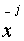are aligned then: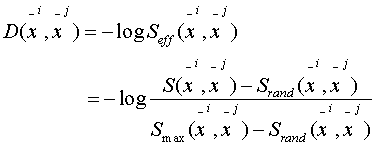where: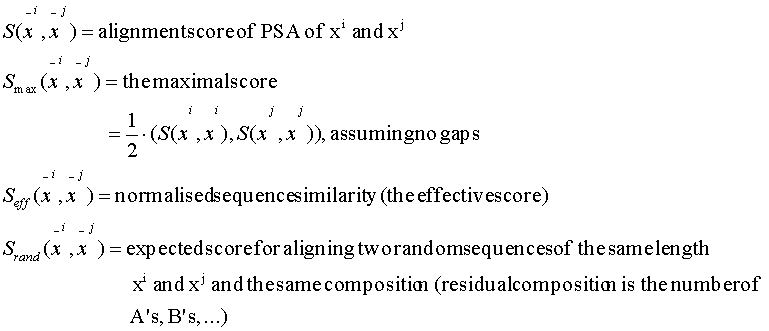In practice: shuffle and compute the sum.

Question: Why do we have another "log" in front of the effective scores?

Answer: The "-log" makes it roughly linear with evolutionary distances.

3. Construct a guide tree from the distance matrix computed at point 1.b). Use a specific clustering algorithm (it will be provided a little bit latter --- phylogenetic trees).
4. Start from the first node that has been added to the guide tree and align the child nodes (using PSA).
5. Repeat step 3. For all other nodes in the order in which they were added to the tree. Do this until all sequences have been aligned.
Question: How to align sequences with groups (MSA) and groups with groups?

Here (for Feng - Doolittle Algorithm)

1. Sequence X vs group Y alignment
Y = {y1, y2, .. , yM}
• Align X to each y from Y using PSA -> gives us a score
• From these values pick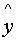that gives us the maximum alignment score with x (i.e. the best alignment)
• Copy all the gaps that have been inserted into all other y from Y\{}
Remark: Once you align strings, they will remain stuck together.

Example:

x .. .. .. .. .. D P V .. .. .. .. .. .... .. .. .. .. .. D - V .. .. .. .. .. .. .. insert a gap

| | | | | | | | | |

y1 .. .. .. .. .. L - V .. .. .. .. .. .. ..

1. Group vs group alignment
X = {x1, x2, .. , xN}

Y = {y1, y2, .. , yM}

• Align each x from X and each y from Y (need quadratic time: N x M)
• Pick the pair (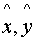) with maximum alignment score
• Copy all gaps inserted into all other x from X\{} and into all other y from Y\{}
Problem: We will align gaps to gaps. How to score them?

Idea: S('-', '-') = 0 i.e. don't penalize and don't add score at all

There is also a problem even if we do it in this way. At a certain stage we align things that will make them somehow incompatible.

Feng - Doolittle Algorithm: after each alignment, replace each gap ('-') with a new character ('X') and it has the property that S('X', *) = 0 Remark: 1) In FD algorithm this implements the rule "once a gap, always a gap".

2) This rule encourages gaps to occur in the same column and "guides" the alignment.

Note: Time complexity of FD is polynomial, i.e. it is in between quadratic and exponential.

Can we do better than FD?

Note: In Feng - Doolittle all alignments are determined by PSA. Why is this not the best idea?

Example:

.. .. .. N .. .. .. .. .. .. .. N .. .. .. ..

.. .. .. N .. .. .. .. .. .. .. V .. .. .. ..

.. .. .. N .. .. .. .. .. .. .. L .. .. .. ..

.. .. .. N .. .. .. .. .. .. .. N .. .. .. ..

.. .. .. P .. .. .. .. .. .. .. P .. .. .. ..

They don't care how many N's are in the other alignments.

Idea: When aligning for groups, we exploit the aggregated position specific information from groups' MSA.

So in fact we want to penalize mismatches of conserved subsequence, more severely than for variable positions.

We want also to lower gap penalties for positions where already lots of gaps occur.

One technique that does this is:

Sequence profile alignment

To do:

1. Align sequences vs profiles

2. Align profile vs profile

Remarks: There are various scoring functions in the literature, most of them being based on SP score and the gap scoring varies widely between different methods.

Here: Assume the linear gap model: F(g) = - gd

1. Align X1 = {x1, x2, .. , xn} to X2 = {xn+1, .. , xN}

Scoring procedure: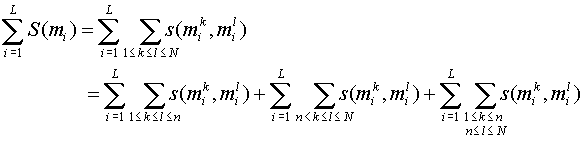Gap scoring:

S('-', ) = S(, '-') = -g

S('-', '-') = 0

Then we can use BLOSUM matrices or something similar.

Note: Sum term 1 and sum term 2 are independent of the alignment of X1 and X2. To get the best alignment we just need to optimize the third sum term. This is analogous to standard PSA if we score columns vs columns when using SP scores and it can be done using a straight-forward generalisation of the standard dynamic programming algorithm for global PSA.

CLUSTALW Program [Thompson, Higgins and Gibson, 1994]

CLUSTALW is one widely used implementation of profile-based progressive multiple alignment.

It is very similar to the Feng - Doolittle algorithm and it works as follows:

1. Construct a distance matrix of all N(N-1)/2 pairs of sequences by pairwise sequence alignment. Then convert the similarity scores to evolutionary distances using a specific model of evolution proposed by Kimura in 1983.

2. Construct a guide-tree from this matrix using a clustering method called neighbor-joining proposed by Saitou and Nei in 1987.

3. Progressively align nodes of the tree in order of decreasing similarity using sequences vs sequences, sequences vs profile and profile vs profile alignments.

We have to add various heuristics to get good accuracy [see Durbin et al., Chapter 6.4, page 148]. Overall, CLUSTALW is a very "handcrafted" algorithm. It would be nice to have something with a better theoretic foundation and comparable or better performance.

New directions:

• Hidden Markov Models (HMM): see Durbin et al., Chapter 6.5
• Gibbs sampling